# RD Sharma Solutions for Class 6 Maths Chapter 3: Whole Numbers

The expert faculty at BYJU’S designs solutions based on the marks allotted for each step of a problem. The primary objective of solving problems is to make the subject easier for students. Each exercise contains examples solved which help students answer the exercise wise problems comfortably. The students are provided with chapter wise solutions in order to help them perform well in the exam.

This Chapter contains concepts on Whole Numbers and their properties which are of greater importance. The solutions which are provided by the faculty are accurate using the methods followed in RD Sharma text book of current CBSE syllabus. RD Sharma Solutions for Class 6 Maths Chapter 3 Whole Numbers are provided here.

## RD Sharma Solutions for Class 6 Maths Chapter 3: Whole Numbers Download PDF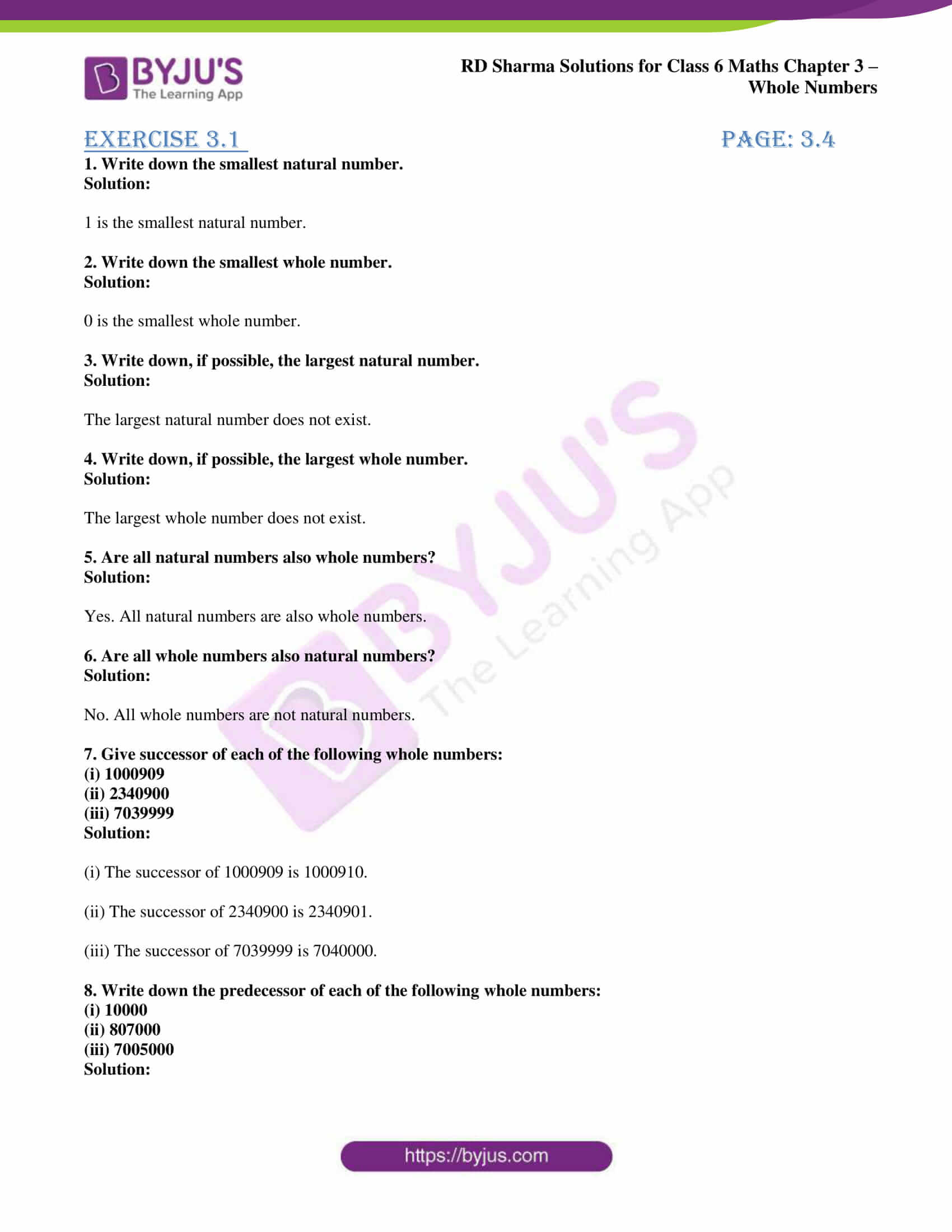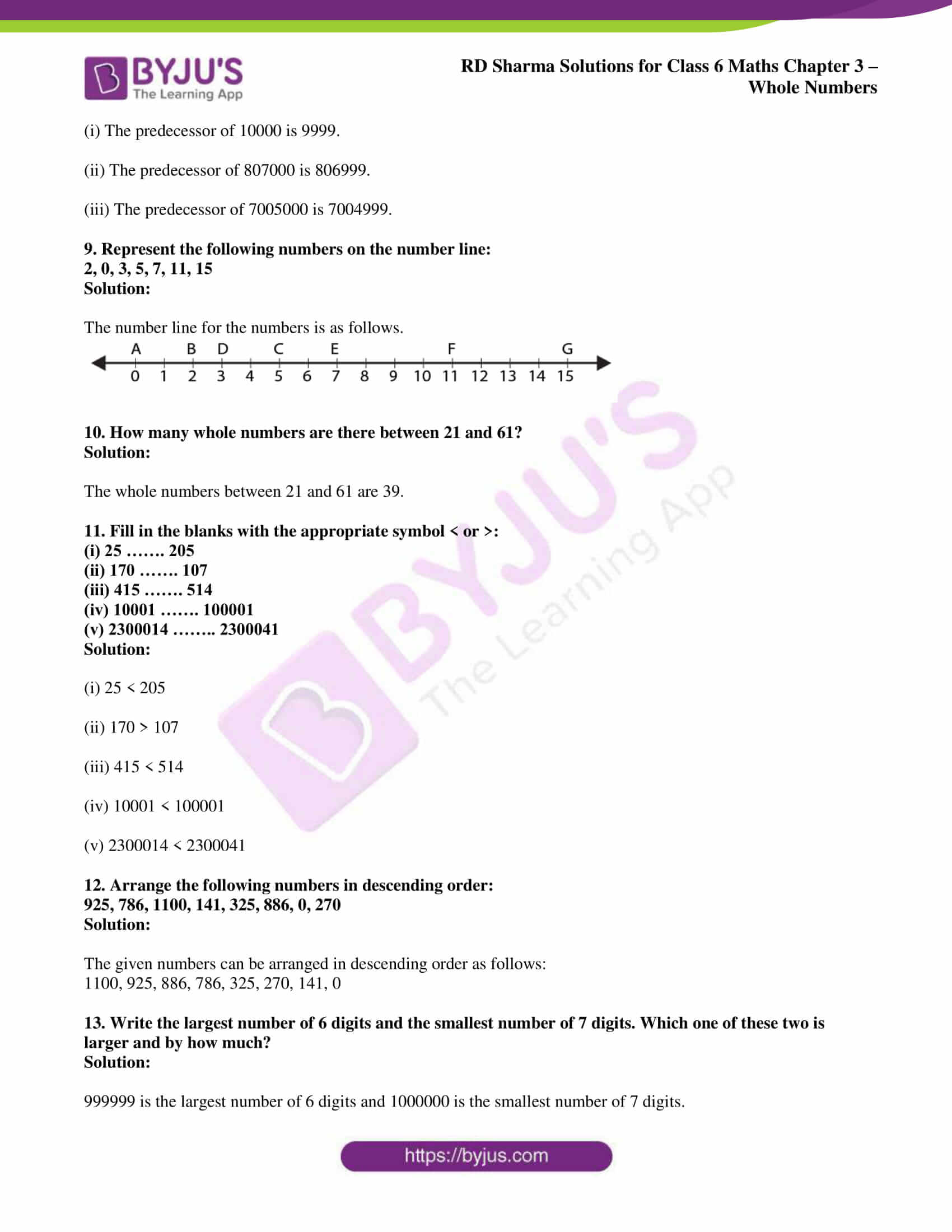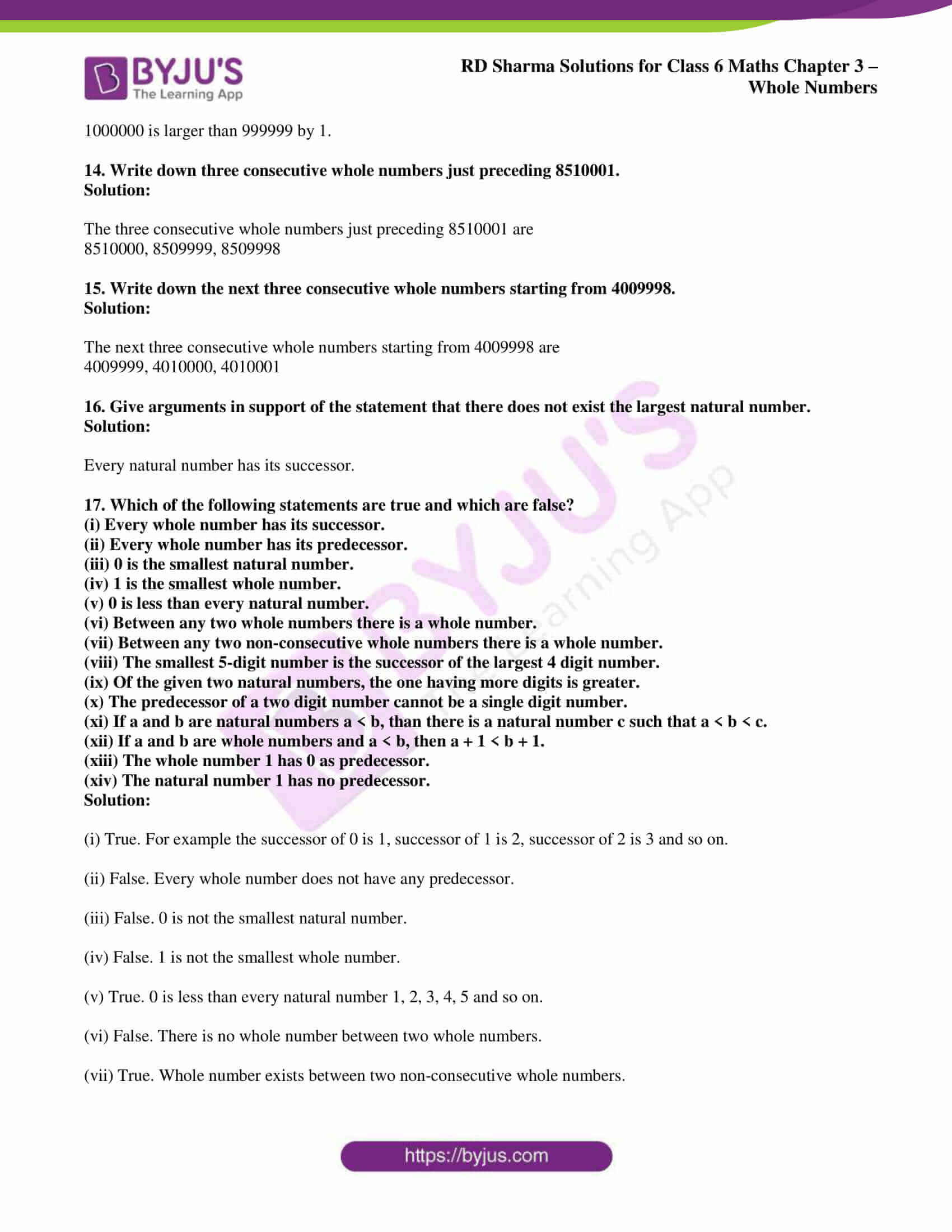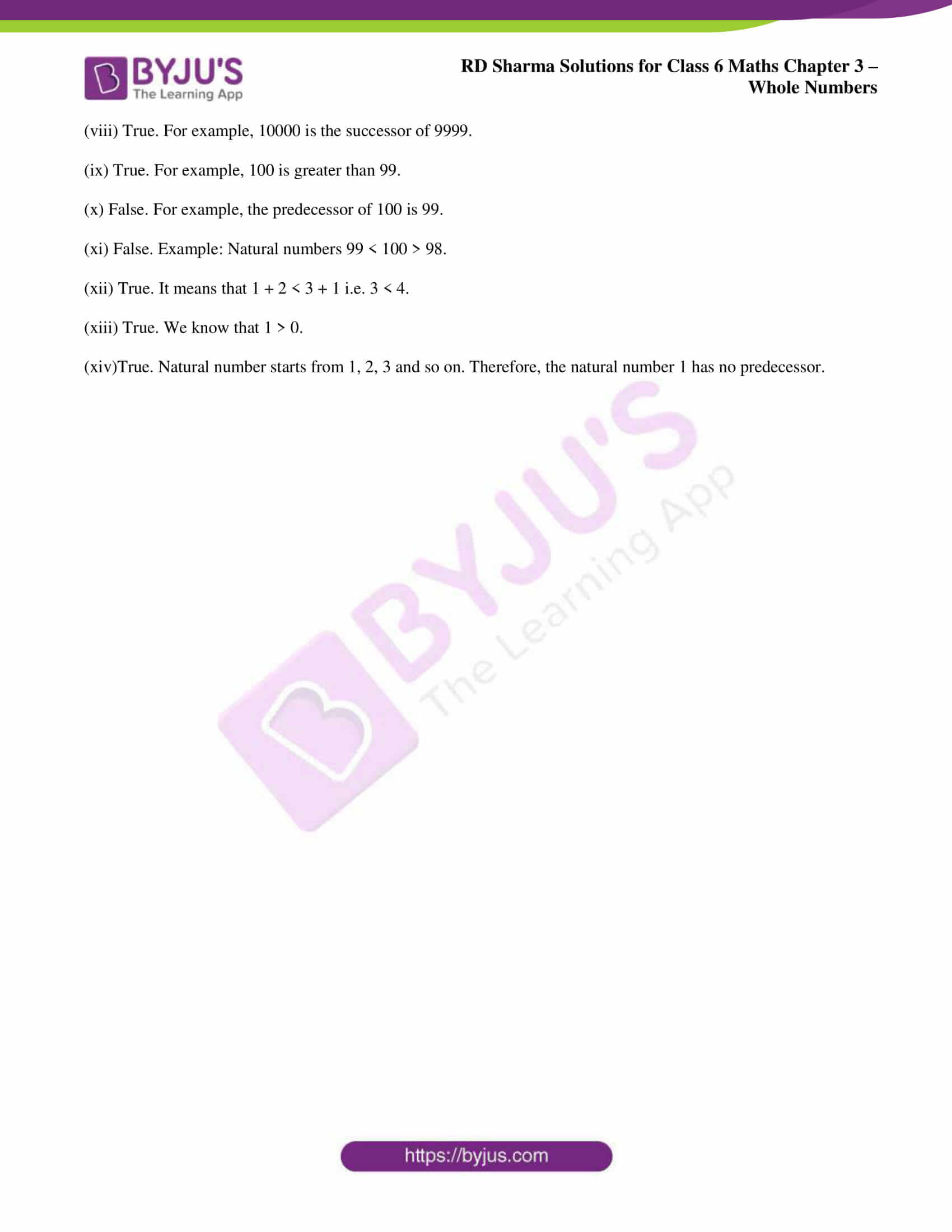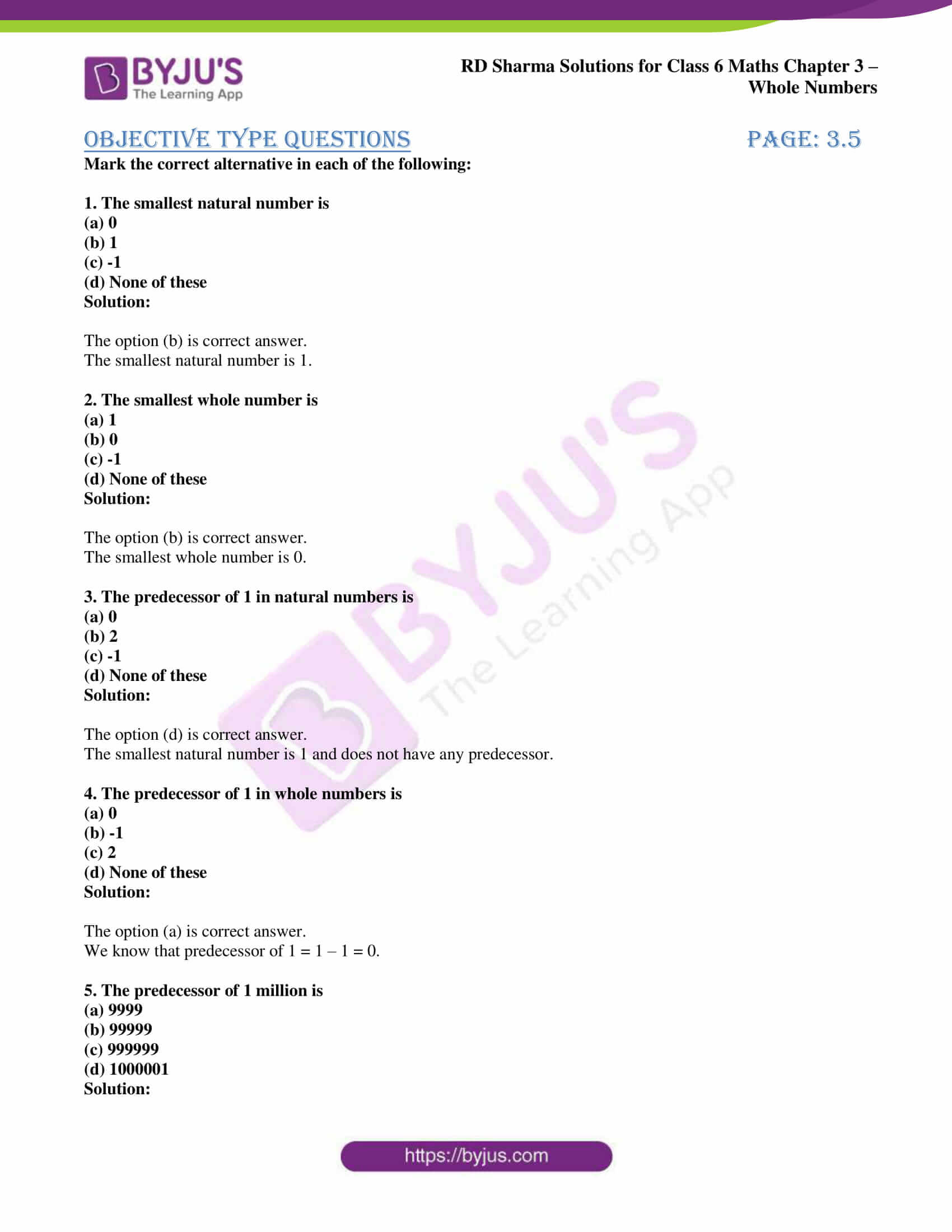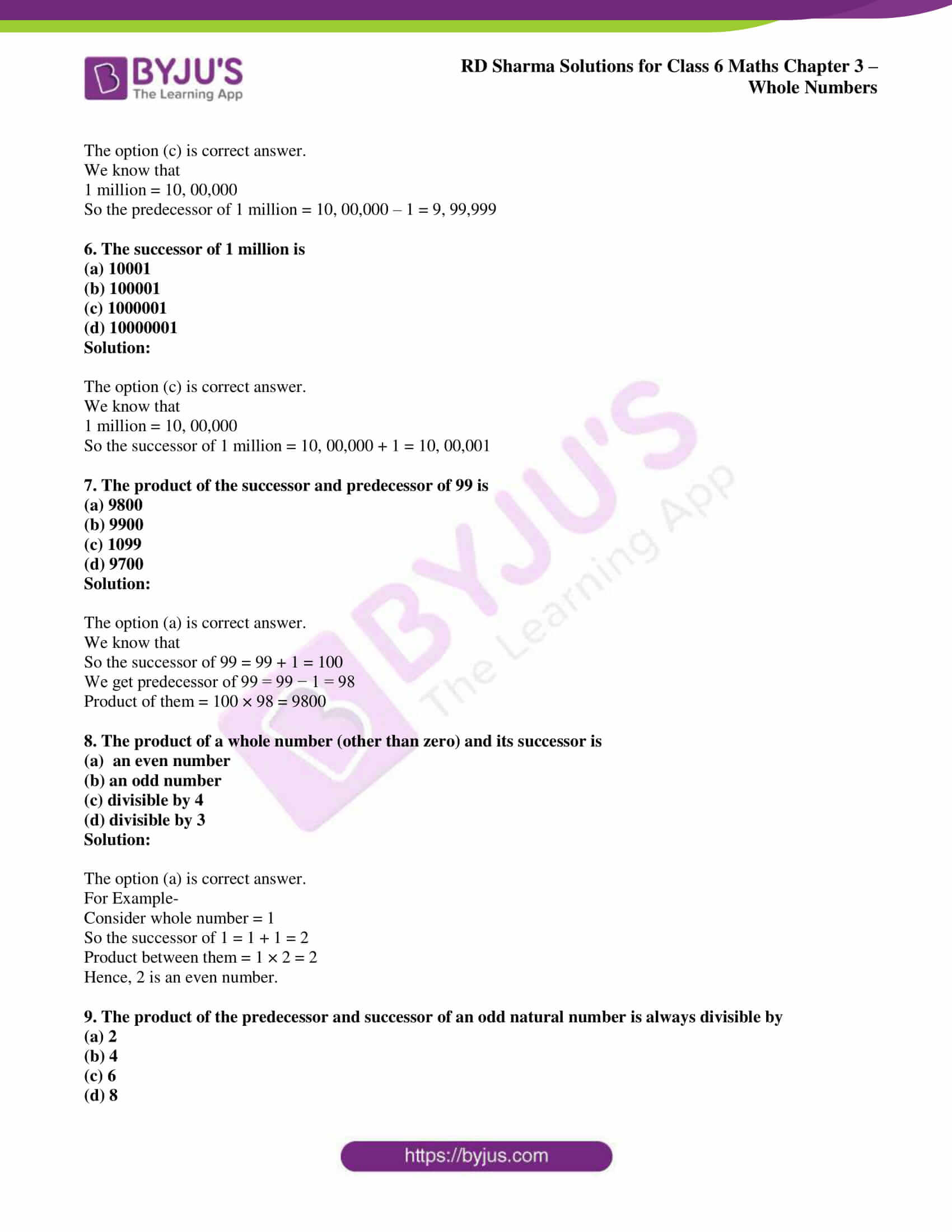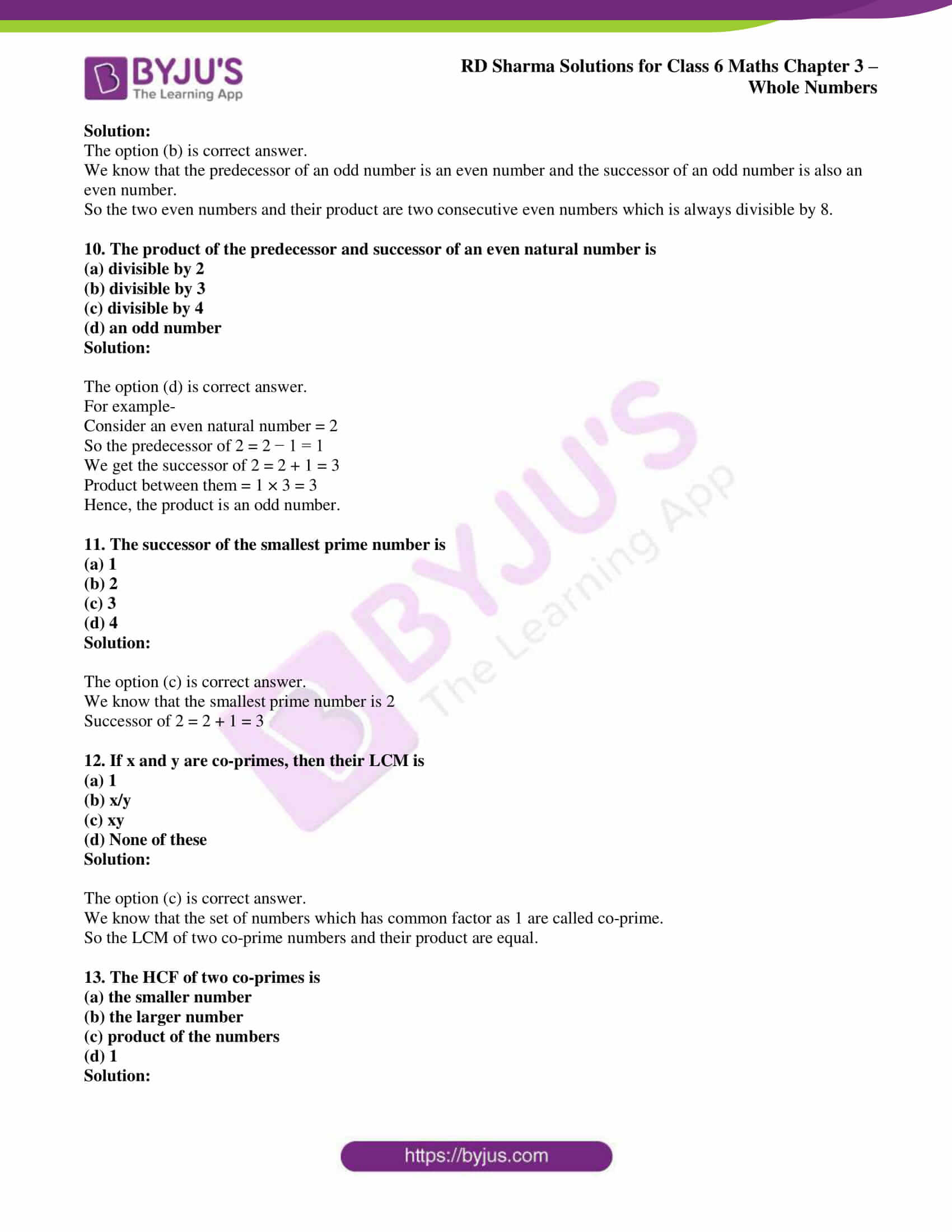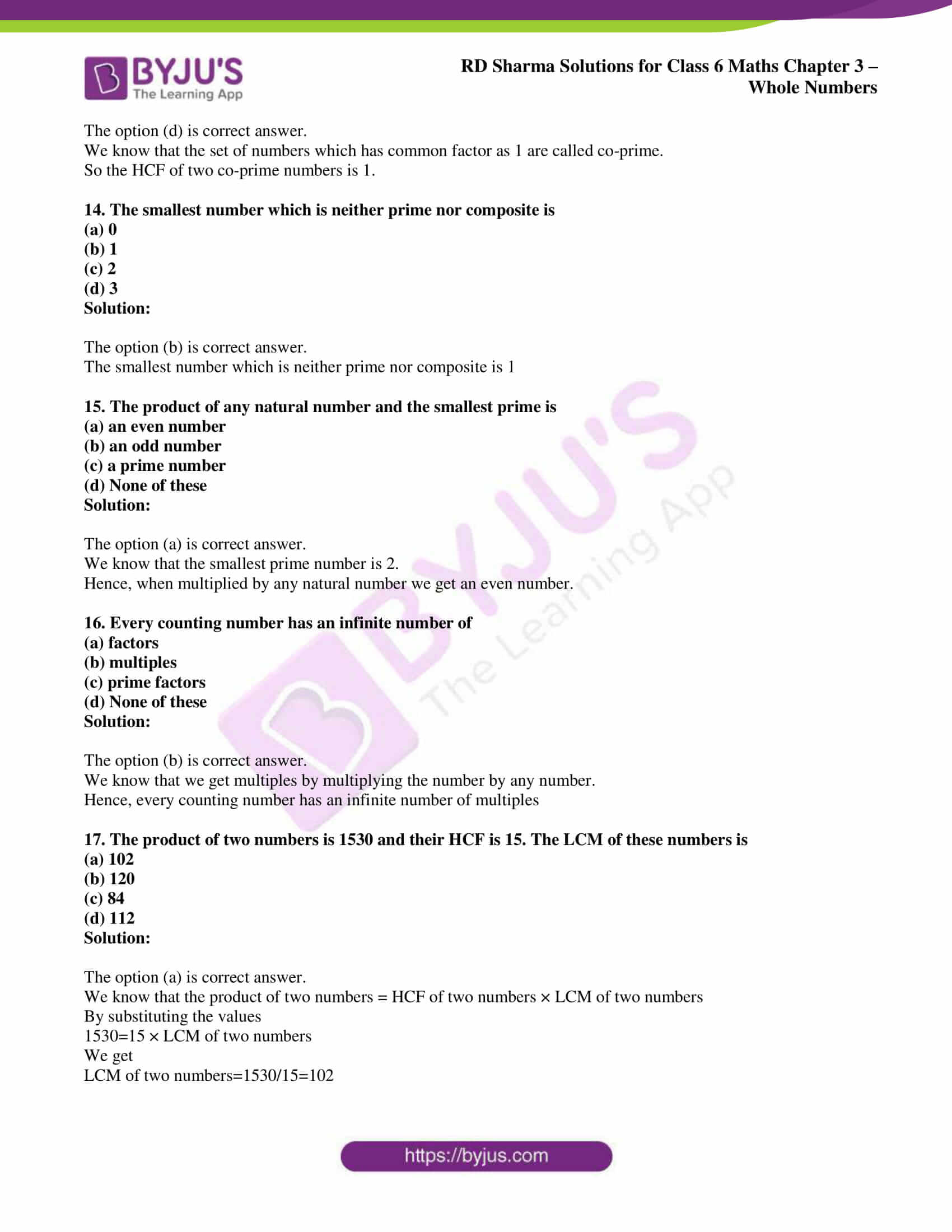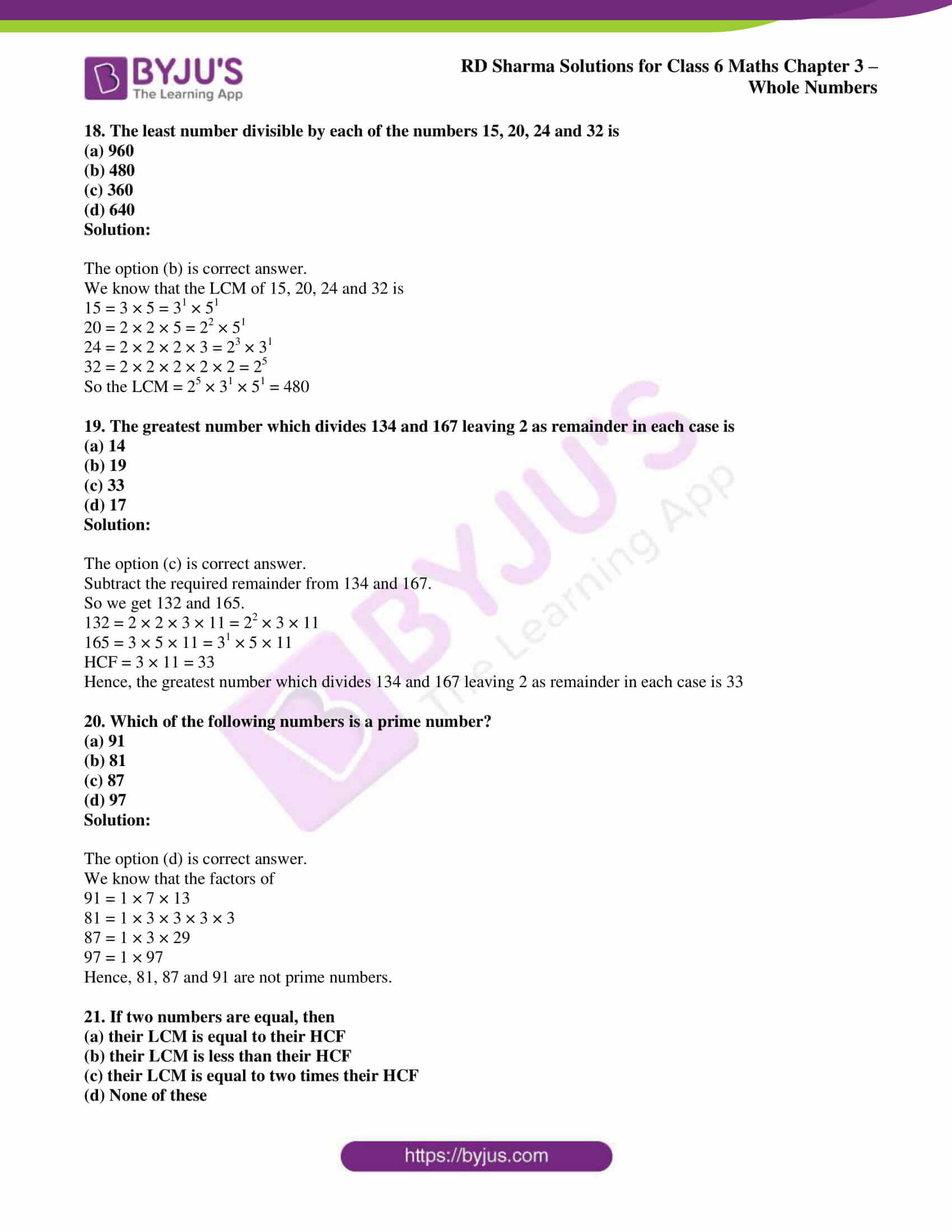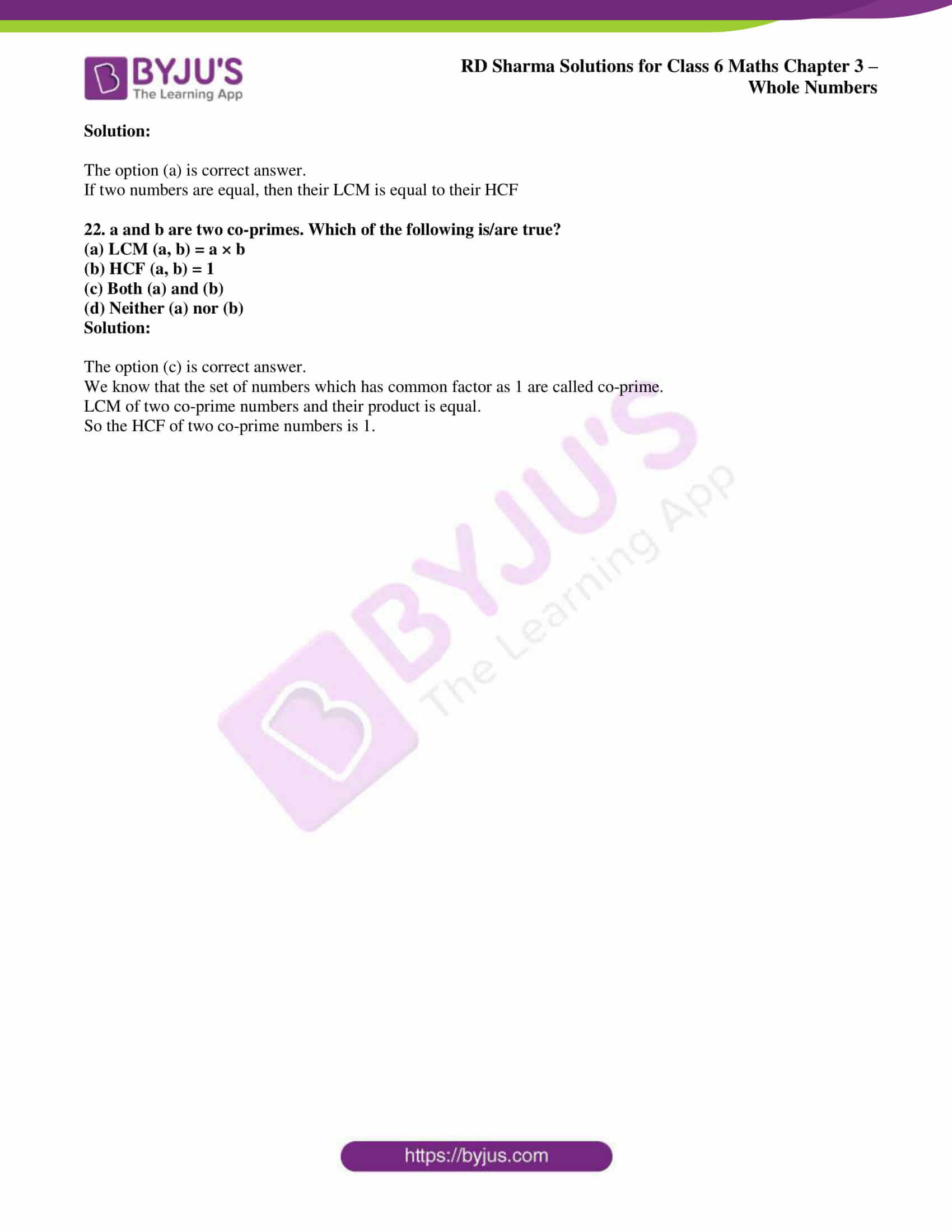## Access answers to Maths RD Sharma Solutions for Class 6 Chapter 3: Whole Numbers

### Exercise 3.1 page: 3.4

1. Write down the smallest natural number.

Solution:

1 is the smallest natural number.

2. Write down the smallest whole number.

Solution:

0 is the smallest whole number.

3. Write down, if possible, the largest natural number.

Solution:

The largest natural number does not exist.

4. Write down, if possible, the largest whole number.

Solution:

The largest whole number does not exist.

5. Are all natural numbers also whole numbers?

Solution:

Yes. All natural numbers are also whole numbers.

6. Are all whole numbers also natural numbers?

Solution:

No. All whole numbers are not natural numbers.

7. Give successor of each of the following whole numbers:

(i) 1000909

(ii) 2340900

(iii) 7039999

Solution:

(i) The successor of 1000909 is 1000910.

(ii) The successor of 2340900 is 2340901.

(iii) The successor of 7039999 is 7040000.

8. Write down the predecessor of each of the following whole numbers:

(i) 10000

(ii) 807000

(iii) 7005000

Solution:

(i) The predecessor of 10000 is 9999.

(ii) The predecessor of 807000 is 806999.

(iii) The predecessor of 7005000 is 7004999.

9. Represent the following numbers on the number line:

2, 0, 3, 5, 7, 11, 15

Solution:

The number line for the numbers is as follows.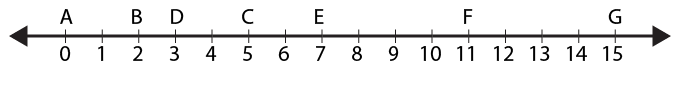10. How many whole numbers are there between 21 and 61?

Solution:

The whole numbers between 21 and 61 are 39.

11. Fill in the blanks with the appropriate symbol < or >:

(i) 25 ……. 205

(ii) 170 ……. 107

(iii) 415 ……. 514

(iv) 10001 ……. 100001

(v) 2300014 …….. 2300041

Solution:

(i) 25 < 205

(ii) 170 > 107

(iii) 415 < 514

(iv) 10001 < 100001

(v) 2300014 < 2300041

12. Arrange the following numbers in descending order:

925, 786, 1100, 141, 325, 886, 0, 270

Solution:

The given numbers can be arranged in descending order as follows:

1100, 925, 886, 786, 325, 270, 141, 0

13. Write the largest number of 6 digits and the smallest number of 7 digits. Which one of these two is larger and by how much?

Solution:

999999 is the largest number of 6 digits and 1000000 is the smallest number of 7 digits.

1000000 is larger than 999999 by 1.

14. Write down three consecutive whole numbers just preceding 8510001.

Solution:

The three consecutive whole numbers just preceding 8510001 are

8510000, 8509999, 8509998

15. Write down the next three consecutive whole numbers starting from 4009998.

Solution:

The next three consecutive whole numbers starting from 4009998 are

4009999, 4010000, 4010001

16. Give arguments in support of the statement that there does not exist the largest natural number.

Solution:

Every natural number has its successor.

17. Which of the following statements are true and which are false?

(i) Every whole number has its successor.

(ii) Every whole number has its predecessor.

(iii) 0 is the smallest natural number.

(iv) 1 is the smallest whole number.

(v) 0 is less than every natural number.

(vi) Between any two whole numbers there is a whole number.

(vii) Between any two non-consecutive whole numbers there is a whole number.

(viii) The smallest 5-digit number is the successor of the largest 4 digit number.

(ix) Of the given two natural numbers, the one having more digits is greater.

(x) The predecessor of a two digit number cannot be a single digit number.

(xi) If a and b are natural numbers a < b, than there is a natural number c such that a < b < c.

(xii) If a and b are whole numbers and a < b, then a + 1 < b + 1.

(xiii) The whole number 1 has 0 as predecessor.

(xiv) The natural number 1 has no predecessor.

Solution:

(i) True. For example the successor of 0 is 1, successor of 1 is 2, successor of 2 is 3 and so on.

(ii) False. Every whole number does not have any predecessor.

(iii) False. 0 is not the smallest natural number.

(iv) False. 1 is not the smallest whole number.

(v) True. 0 is less than every natural number 1, 2, 3, 4, 5 and so on.

(vi) False. There is no whole number between two whole numbers.

(vii) True. Whole number exists between two non-consecutive whole numbers.

(viii) True. For example, 10000 is the successor of 9999.

(ix) True. For example, 100 is greater than 99.

(x) False. For example, the predecessor of 100 is 99.

(xi) False. Example: Natural numbers 99 < 100 > 98.

(xii) True. It means that 1 + 2 < 3 + 1 i.e. 3 < 4.

(xiii) True. We know that 1 > 0.

(xiv)True. Natural number starts from 1, 2, 3 and so on. Therefore, the natural number 1 has no predecessor.

### Objective Type Questions PAGE: 3.5

Mark the correct alternative in each of the following:

1. The smallest natural number is
(a) 0
(b) 1
(c) -1
(d) None of these

Solution:

The option (b) is correct answer.

The smallest natural number is 1.

2. The smallest whole number is
(a) 1
(b) 0
(c) -1
(d) None of these

Solution:

The option (b) is correct answer.

The smallest whole number is 0.

3. The predecessor of 1 in natural numbers is
(a) 0
(b) 2
(c) -1
(d) None of these

Solution:

The option (d) is correct answer.

The smallest natural number is 1 and does not have any predecessor.

4. The predecessor of 1 in whole numbers is
(a) 0
(b) -1
(c) 2
(d) None of these

Solution:

The option (a) is correct answer.

We know that predecessor of 1 = 1 – 1 = 0.

5. The predecessor of 1 million is
(a) 9999
(b) 99999
(c) 999999
(d) 1000001

Solution:

The option (c) is correct answer.

We know that

1 million = 10, 00,000

So the predecessor of 1 million = 10, 00,000 – 1 = 9, 99,999

6. The successor of 1 million is
(a) 10001
(b) 100001
(c) 1000001
(d) 10000001

Solution:

The option (c) is correct answer.

We know that

1 million = 10, 00,000

So the successor of 1 million = 10, 00,000 + 1 = 10, 00,001

7. The product of the successor and predecessor of 99 is
(a) 9800
(b) 9900
(c) 1099
(d) 9700

Solution:

The option (a) is correct answer.

We know that

So the successor of 99 = 99 + 1 = 100

We get predecessor of 99 = 99 − 1 = 98

Product of them = 100 × 98 = 9800

8. The product of a whole number (other than zero) and its successor is
(a)  an even number
(b) an odd number
(c) divisible by 4
(d) divisible by 3

Solution:

The option (a) is correct answer.

For Example-

Consider whole number = 1

So the successor of 1 = 1 + 1 = 2

Product between them = 1 × 2 = 2

Hence, 2 is an even number.

9. The product of the predecessor and successor of an odd natural number is always divisible by
(a) 2
(b) 4
(c) 6
(d) 8

Solution:

The option (b) is correct answer.

We know that the predecessor of an odd number is an even number and the successor of an odd number is also an even number.

So the two even numbers and their product are two consecutive even numbers which is always divisible by 8.

10. The product of the predecessor and successor of an even natural number is
(a) divisible by 2
(b) divisible by 3
(c) divisible by 4
(d) an odd number

Solution:

The option (d) is correct answer.

For example-

Consider an even natural number = 2

So the predecessor of 2 = 2 − 1 = 1

We get the successor of 2 = 2 + 1 = 3

Product between them = 1 × 3 = 3

Hence, the product is an odd number.

11. The successor of the smallest prime number is
(a) 1
(b) 2
(c) 3
(d) 4

Solution:

The option (c) is correct answer.

We know that the smallest prime number is 2

Successor of 2 = 2 + 1 = 3

12. If x and y are co-primes, then their LCM is
(a) 1
(b) x/y
(c) xy
(d) None of these

Solution:

The option (c) is correct answer.

We know that the set of numbers which has common factor as 1 are called co-prime.

So the LCM of two co-prime numbers and their product are equal.

13. The HCF of two co-primes is
(a) the smaller number
(b) the larger number
(c) product of the numbers
(d) 1

Solution:

The option (d) is correct answer.

We know that the set of numbers which has common factor as 1 are called co-prime.

So the HCF of two co-prime numbers is 1.

14. The smallest number which is neither prime nor composite is
(a) 0
(b) 1
(c) 2
(d) 3

Solution:

The option (b) is correct answer.

The smallest number which is neither prime nor composite is 1

15. The product of any natural number and the smallest prime is
(a) an even number
(b) an odd number
(c) a prime number
(d) None of these

Solution:

The option (a) is correct answer.

We know that the smallest prime number is 2.

Hence, when multiplied by any natural number we get an even number.

16. Every counting number has an infinite number of
(a) factors
(b) multiples
(c) prime factors
(d) None of these

Solution:

The option (b) is correct answer.

We know that we get multiples by multiplying the number by any number.

Hence, every counting number has an infinite number of multiples

17. The product of two numbers is 1530 and their HCF is 15. The LCM of these numbers is
(a) 102
(b) 120
(c) 84
(d) 112

Solution:

The option (a) is correct answer.

We know that the product of two numbers = HCF of two numbers × LCM of two numbers

By substituting the values

1530=15 × LCM of two numbers

We get

LCM of two numbers=1530/15=102

18. The least number divisible by each of the numbers 15, 20, 24 and 32 is
(a) 960
(b) 480
(c) 360
(d) 640

Solution:

The option (b) is correct answer.

We know that the LCM of 15, 20, 24 and 32 is

15 = 3 × 5 = 31 × 51

20 = 2 × 2 × 5 = 22 × 51

24 = 2 × 2 × 2 × 3 = 23 × 31

32 = 2 × 2 × 2 × 2 × 2 = 25

So the LCM = 25 × 31 × 51 = 480

19. The greatest number which divides 134 and 167 leaving 2 as remainder in each case is
(a) 14
(b) 19
(c) 33
(d) 17

Solution:

The option (c) is correct answer.

Subtract the required remainder from 134 and 167.

So we get 132 and 165.

132 = 2 × 2 × 3 × 11 = 22 × 3 × 11

165 = 3 × 5 × 11 = 31 × 5 × 11

HCF = 3 × 11 = 33

Hence, the greatest number which divides 134 and 167 leaving 2 as remainder in each case is 33

20. Which of the following numbers is a prime number?
(a) 91
(b) 81
(c) 87
(d) 97

Solution:

The option (d) is correct answer.

We know that the factors of

91 = 1 × 7 × 13

81 = 1 × 3 × 3 × 3 × 3

87 = 1 × 3 × 29

97 = 1 × 97

Hence, 81, 87 and 91 are not prime numbers.

21. If two numbers are equal, then
(a) their LCM is equal to their HCF
(b) their LCM is less than their HCF
(c) their LCM is equal to two times their HCF
(d) None of these

Solution:

The option (a) is correct answer.

If two numbers are equal, then their LCM is equal to their HCF

22. a and b are two co-primes. Which of the following is/are true?
(a) LCM (a, b) = a × b
(b) HCF (a, b) = 1
(c) Both (a) and (b)
(d) Neither (a) nor (b)

Solution:

The option (c) is correct answer.

We know that the set of numbers which has common factor as 1 are called co-prime.

LCM of two co-prime numbers and their product is equal.

So the HCF of two co-prime numbers is 1.

### RD Sharma Solutions for Class 6 Maths Chapter 3: Whole Numbers

Chapter 3, Whole Numbers, has 1 exercise which describes the properties of whole numbers and methods followed in solving problems. The major concepts which are explained in RD Sharma Solutions Chapter 3 are listed down:

• Introduction
• Natural Numbers
• Properties of Natural Numbers
• The number zero
• Whole Numbers
• Successor and Predecessor of a Whole Number
• Representation of Whole Numbers on Number Line
• Properties of Whole Numbers

### Chapter brief of RD Sharma Solutions Class 6 Maths Chapter 3 – Whole Numbers

The solutions of chapter wise problems are prepared by subject matter experts based on the understanding capacity of students. The PDF of chapter wise solutions can be used by the students as a vital resource to improve their problem solving abilities. Each question of the exercise is solved using various methods to make them easily understandable by the students.

We know that the numbers from 0, 1, 2, 3 …are whole numbers, where a whole number is either zero or natural number. We use whole numbers in our daily life to count things and to obtain the required quantity. So, students can use the PDF to obtain further knowledge about concepts which are explained in this Chapter.